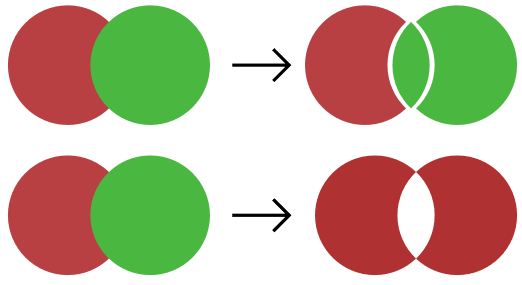# Divide two shapes in three

I cannot find a way to divide two shapes into three. What I mean, is that if you have two shapes partly covering each other then how should I divide them into three shapes, two of which are the parts which were not covered by anything, and third the intersection of those two. See figure 1.

There is Unite, Intersect, and Subtract. Divide the another important shape manipulation action. Another missing is shape manipulation action is Combine, which only combines everything except the intersection. Figure 2.